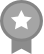`Hey!! welcome to my Wattpad.. first of all.. sorry for my english because it's not my first language.. and i hope you will like it.. 💙잘 부탁드립니다💙=•=•=•=•=•=•=•=•=•=•=•=•=•=•=•=•=•=•=•=Hey There✌i'm Lvie Vivy✌Kpop fans ✌=•=•=•=•=•=•=•=•=•=•=•=•=•=•=•=•=•=•=•=BTS💙 SVT💙 BP💙 IU💙 TY💙=•=•=•=•=•=•=•=•=•=•=•=•=•=•=•=•=•=•=•=`
• JoinedFebruary 13, 2018

Following

Last MessageSunshineCruisee May 11, 2020 03:07PM
`Omgeee.. hey .. thank you so much for giving so much love for my books.. i really glad to know that you like it.. nice to meet you btw.. First of all, i'm so sorry .. because i don't do any of updat...`

Stories by Sunshine Cruise✔️RUDE HUSBAND [J.J.K] (COMPLETE)
Theme: Romance / Adult / Love / Married Content: +18 Actor: Jeon JungKook BTS you have to married with someon...#5 in loveromance See all rankingsMy Chimmy [P.J.M] (ON GOING)
Theme: Romance / Adult / Love / Sadness Content: +18 Actor: Park Jimin BTS Loving him is precious thing have...
+6 more